### Showing videos fromEddie Woo with a total of 4,051 videos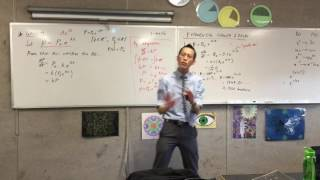11:37
Exponential Growth and Decay (3 of 4: Working through an introductory example of Exponential Growth)
3y0m ago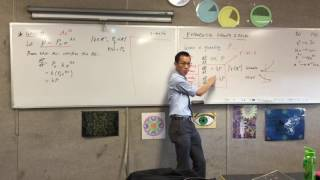11:09
Exponential Growth and Decay (2 of 4: Satisfying the DE by integration/differentiation)
3y0m ago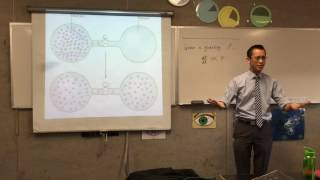10:46
Exponential Growth and Decay (1 of 4: Representing growth in proportion to size of population)
3y0m ago10:01
Perimeter (2 of 2: Calculating the Perimeter of shapes with circular curvature)
3y0m ago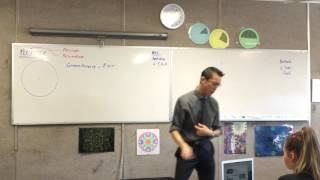05:14
Perimeter (1 of 2: Properties of a Circle & the Circumference and area formulae)
3y0m ago07:12
Percentage Change (3 of 3: Implications of percentage change & what to divide change over)
3y0m ago06:08
Percentage Change (2 of 3: Using Calculator to find percentage change & some introductory question)
3y0m ago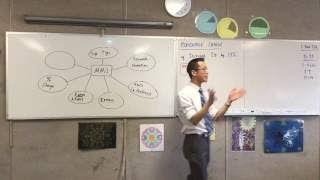10:43
Percentage Change (1 of 3: Introducing uses of Percentage change and how it fits with MM1 topics)
3y0m ago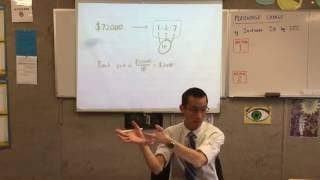08:00
General 2 Quiz (2 of 2: Ratios and Rate & how to calculate ratios)
3y0m ago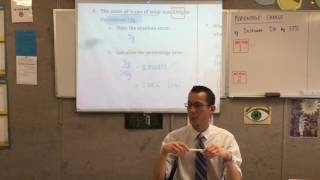08:27
General 2 Quiz (1 of 2: Algebra Manipulation, Scientific Notation & Percentage & Absolute Error)
3y0m ago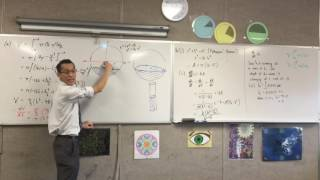05:00
Rates of Change (4 of 4: Integrating to find the height of the 'puddle')
3y0m ago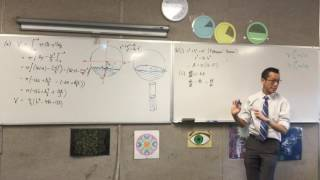07:24
Rates of Change (3 of 4: Using Chain Rule to find the change of height over time)
3y0m ago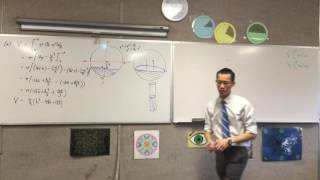05:40
Rates of Change (2 of 4: Using a second diagram to find r in terms of h for a SA relation)
3y0m ago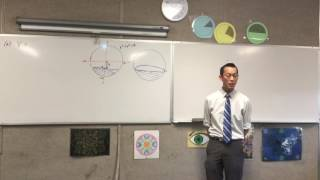07:25
Rates of Change (1 of 4: Finding the Volume of an unknown height of water with a diagram)
3y0m ago08:06
Rates of Change: Integration (4 of 4: Finding time to release specific amount of water)
3y0m ago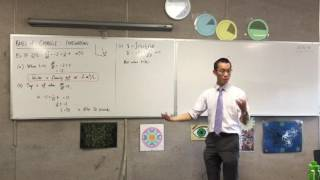06:07
Rates of Change: Integration (3 of 4: Using the Volume Function to find time of certain events)
3y0m ago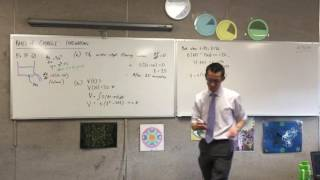08:20
Rates of Change: Integration (2 of 4: Integrating to find v(t) and using it to find Initial Volume)
3y0m ago05:48
Rates of Change: Integration (1 of 4: Understanding information from question)
3y0m ago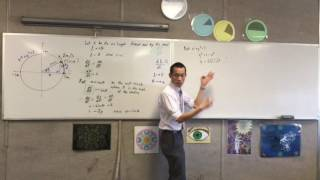09:47
Harder Rates of Change Question (2 of 2: Using Differentiation and Chain Rule to find solution)
3y0m ago10:44
Harder Rates of Change Question (1 of 2: Extrapolating information from question to find solution)
3y0m ago09:01
Proof by Mathematical Induction (2 of 2: Using expression that is more restrictive to prove n=k+1)
3y0m ago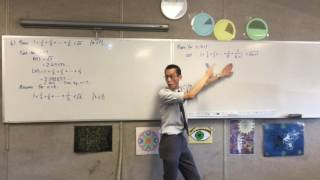05:20
Proof by Mathematical Induction (1 of 2: Getting a statement to prove for n=k+1)
3y0m ago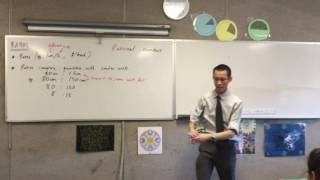12:30
Ratios (Connecting Rates with Ratios and Simplifying to compare similar results)
3y0m ago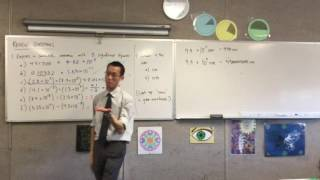05:28
Rounding and Approximation (2 of 2: Reviewing Unit Conversions)
3y0m ago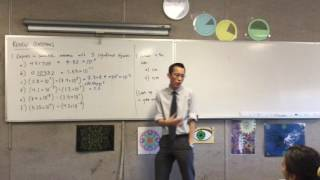09:59
Rounding and Approximation (1 of 2: Reviewing Rounding)
3y0m ago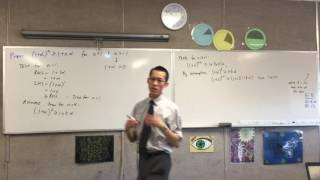13:08
Mathematical Induction (Harder Inequalities Proof by mathematical induction)
3y0m ago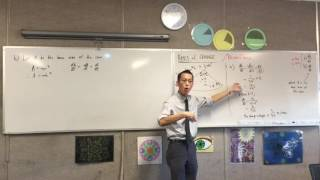06:39
Rates of Change (3 of 3: Using Chain Rule to solve for the rate of change in area)
3y0m ago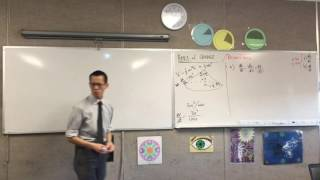09:09
Rates of Change (2 of 3: Using Volume, Area and other formulae and chain rule to find related rates)
3y0m ago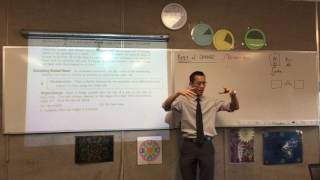07:56
Rates of Change (1 of 3: Calculating Related Rates)
3y0m ago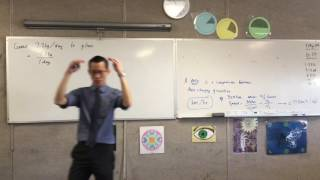03:56
Rates (3 of 3: Converting one type of rate to another)
3y0m ago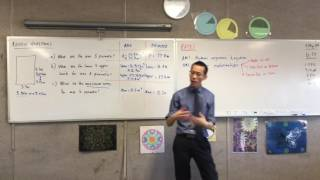07:06
Rates (2 of 3: Introduction to Rates and how to get rates with given measurements)
3y0m ago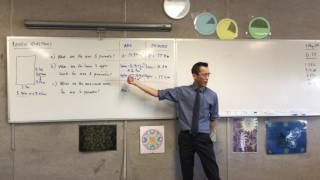08:48
Rates (1 of 3: Reviewing errors in measurement; max and minimum possible values)
3y0m ago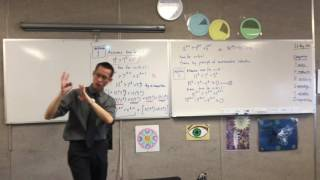10:16
Mathematical Induction (3 of 3: A different approach - rearranging the inequality)
3y0m ago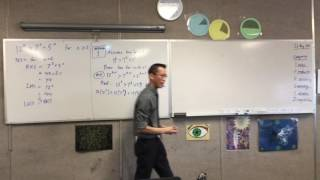08:25
Mathematical Induction (2 of 3: Assumption step and Proving inequality)
3y0m ago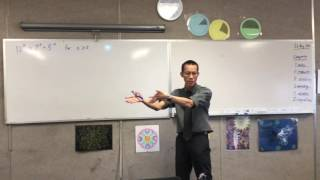06:54
Mathematical Induction (1 of 3: Outlining categories of induction, start of inequalities question)
3y0m ago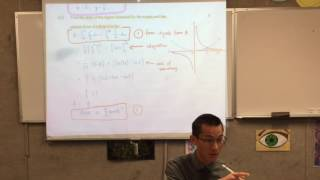12:28
Extension I Quiz (Graphing, Area between curves, Differentiation and Induction)
3y0m ago08:25
Translating Words to Algebra (2 of 2: Converting words into mathematical terms for calculations)
3y0m ago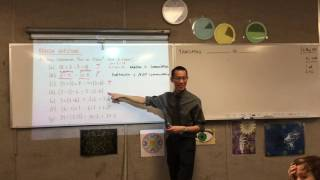09:21
Translating Words to Algebra (1 of 2: Reviewing Commutative, Associative & Distributive Numbers)
3y0m ago14:08
Introduction to Mathematical Induction (2 of 2: A straightforward example proof)
3y0m ago06:54
Introduction to Mathematical Induction (1 of 2: Two Different kinds of Logic)
3y0m ago05:46
Introduction to Algebra (2 of 2: Outlining Abbreviations in Algebra, Simplifying algebraic terms)
3y0m ago09:08
Introduction to Algebra (1 of 2: What Happens when you don't know a number which you need to find?)
3y0m ago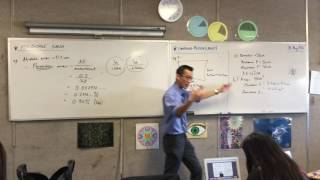09:18
Errors in Measurement II (2 of 2: Working with Ranges when Combining measurements)
3y0m ago08:19
Errors in Measurement II (1 of 2: Introduction to Percentage Errors and an Introductory Example)
3y0m ago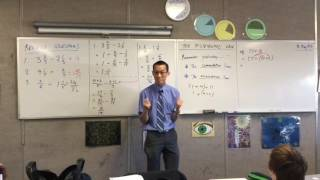07:50
The Distributive Law (2 of 2: Linking the Commutative Law and Associative Law together)
3y0m ago08:46
The Distributive Law (1 of 2: Using two approaches to subtract fractions & Multiply Fractions)
3y0m ago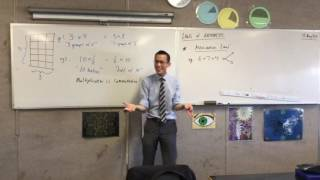03:32
Laws of Arithmetic (3 of 3: The Associative Law)
3y0m ago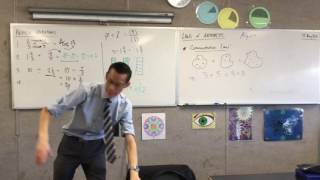08:42
Laws of Arithmetic (2 of 3: The Commutative Law)
3y0m ago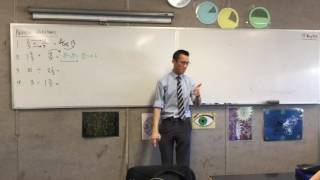08:34
Laws of Arithmetic (1 of 3: Computation with Fractions)
3y0m ago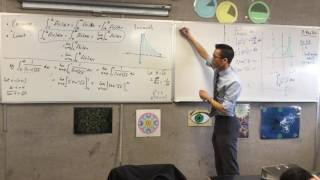08:23
Properties of Definite Integrals (6 of 6: Using Integration by substitution to evaluate integral)
3y0m ago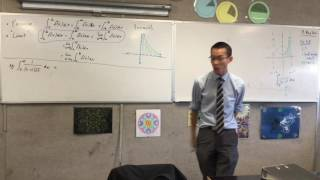05:55
Properties of Definite Integrals (5 of 6: Using piecewise and limit properties for famous result)
3y0m ago07:31
Properties of Definite Integrals (4 of 6: Outlining the Piecewise and "Limit" Properties)
3y0m ago10:44
Properties of Definite Integrals (3 of 6: Using the Reflective Property to solve an integral)
3y0m ago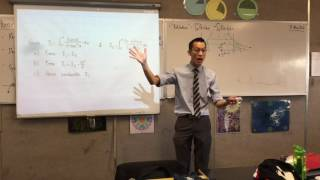09:14
Properties of Definite Integrals (2 of 6: Outlining the 'Reflective' Property)
3y0m ago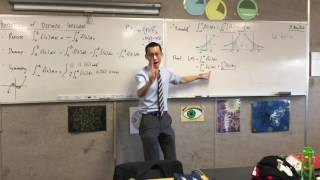12:36
Properties of Definite Integrals (1 of 6: "Round Off" Property of definite integrals)
3y0m ago08:16
Limit of Reading (Rounding to an accuracy within the limit of reading)
3y0m ago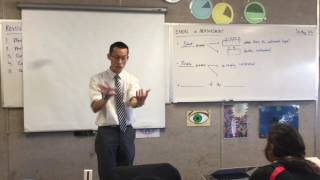08:36
Errors in Measurement (2 of 2: Outline of Start & Scale errors and Instrument Limitations)
3y0m ago03:34
Errors in Measurement (1 of 2: Reviewing significant figures and unit conversion)
3y0m ago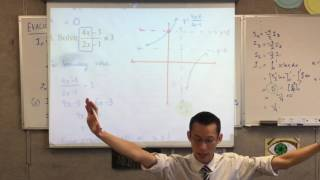12:28
Extension I Quiz (Differentiation of Trig, Logarithms, Integration and Parametrics of Parabola)
3y0m ago07:00
Properties of Definite Integrals (Outline of the Reverse, Dummy and Symmetry properties)
3y0m ago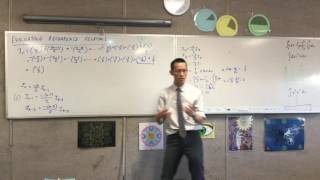10:09
Evaluating Recurrence Relations (4 of 4: Finding a term free of integrals)
3y0m ago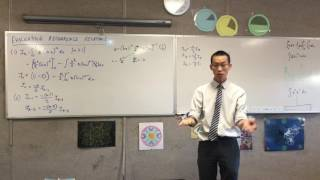06:48
Evaluating Recurrence Relations (3 of 4: Using Recurring Pattern to group Relation in a series)
3y0m ago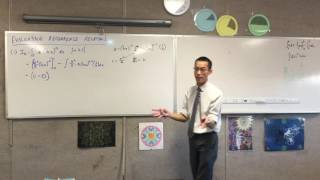09:11
Evaluating Recurrence Relations (2 of 4: Using Integration of parts to build recurring pattern)
3y0m ago05:29
Evaluating Recurrence Relations (1 of 4: When do you apply Recurrence Relations?)
3y0m ago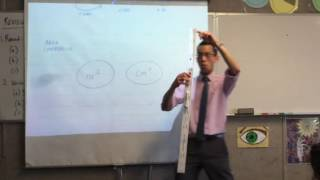06:09
Metric Units of Measurement (3 of 3: Converting between lengths and areas)
3y0m ago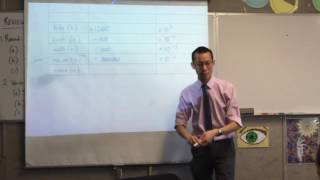06:13
Metric Units of Measurement (2 of 3: Different prefixes and their respective positions)
3y0m ago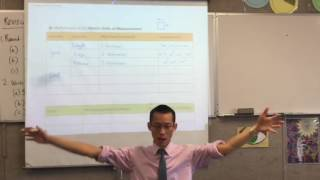10:46
Metric Units of Measurement (1 of 3: Overview of various metric units)
3y0m ago04:57
Rounding and Approximation (Reviewing Significant Figures)
3y0m ago04:07
Scientific Notation (2 of 2: Role of the Scientific Calculator in Scientific Notation)
3y0m ago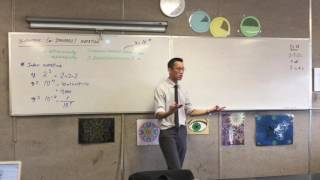08:53
Scientific (Standard) Notation (1 of 2: Simplifying large numbers with scientific notation)
3y0m ago